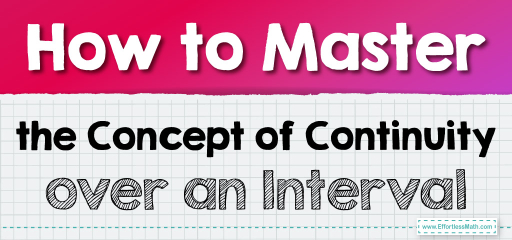# How to Master the Concept of Continuity over an Interval

Embarking on a mathematical journey often involves traversing the landscapes of functions and their graphs, where the notion of continuity plays a crucial role akin to a continuous, unobstructed path in the realm of calculus. The concept of continuity over an interval is particularly intriguing as it dictates whether a function can be graphed as a seamless curve or if it encounters abrupt changes such as jumps, breaks, or holes. To navigate these continuous paths, one must delve into the intricacies of limits, function values, and the behavior of functions as they approach specific points. So, join us as we unravel the conditions that characterize a function's uninterrupted journey across an interval and learn how to identify and classify the various forms of discontinuities.## Step-by-step Guide to Master the Concept of Continuity over an Interval

Here is a step-by-step guide to mastering the concept of continuity over an Interval:

### Step 1: Understand the Basic Concept of an Interval

An interval in mathematics refers to a range of numbers between two specific values. It can come in several types:

• Open Interval $$(a,b)$$: This includes all the numbers between $$a$$ and $$b$$, but not $$a$$ or $$b$$ themselves.
• Closed Interval $$[a,b]$$: This includes all the numbers between $$a$$ and $$b$$, including $$a$$ and $$b$$.
• Semi-Open Interval: It can either include the lower bound but not the upper bound $$[a,b)$$, or include the upper bound but not the lower bound $$(a,b]$$.

### Step 2: Know What It Means for a Function to be Continuous at a Point

Before considering an interval, remember that a function is continuous at a point $$c$$ if:

1. $$f(c)$$ is defined.
2. The limit of $$f(x)$$ as $$x$$ approaches $$c$$ exists.
3. The limit of $$f(x)$$ as $$x$$ approaches $$c$$ is equal to $$f(c)$$.

### Step 3: Extend This Understanding to an Entire Interval

A function $$f(x)$$ is said to be continuous over an interval if it meets the conditions for continuity (as described in Step $$2$$) at every point within that interval.

### Step 4: Verify Continuity Over an Open Interval $$(a,b)$$

To check if a function is continuous over an open interval:

1. Make sure that for every point $$c$$ in the interval $$(a,b)$$, the function $$f(c)$$ is defined.
2. Confirm that the limit of $$f(x)$$ as $$x$$ approaches any point $$c$$ within the interval exists and is equal to $$f(c)$$.

You do not need to consider the endpoints $$a$$ and $$b$$ for open intervals, as they are not included in the interval itself.

### Step 5: Verify Continuity Over a Closed Interval $$[a,b]$$

For a function to be continuous over a closed interval:

1. Ensure the function satisfies all conditions for continuity at every point in the interval $$(a, b)$$.
2. Specifically, check continuity at the endpoints $$a$$ and $$b$$. This means checking that the limits from the right at $$a$$ and from the left at $$b$$ exist and that they equal $$f(a)$$ and $$f(b)$$ respectively.

### Step 6: Check Continuity Over Semi-Open Intervals

For semi-open intervals such as $$[a,b)$$ or $$(a,b]$$, follow the same steps as for the closed interval, but you only need to check the endpoint that is included.

### Step 7: Look for Gaps, Jumps, and Holes

• Gaps and Holes: If there’s a value of $$x$$ within the interval where the function doesn’t have a real number value (a hole if the limit exists, a gap if it does not), the function isn’t continuous.
• Jumps: If there’s a sudden change in the value of the function (a jump), it’s not continuous.

### Step 8: Utilize Graphical Analysis

Often, a graphical look at the function can help. A function that’s continuous over an interval can be represented by a single, unbroken curve over that interval.

### Step 9: Consider Special Cases

Sometimes, a function can be made continuous by redefining it at points of discontinuity (removable discontinuities). Be aware of these when considering continuity over an interval.

### Step 10: Conclude Continuity Over the Interval

After examining all the points within the interval, if the function meets the continuity criteria at each point, you can confidently state that the function is continuous over that interval.

By following this step-by-step guide, you can determine whether a function exhibits the smooth and uninterrupted behavior required to be considered continuous over any given interval.

### What people say about "How to Master the Concept of Continuity over an Interval - Effortless Math: We Help Students Learn to LOVE Mathematics"?

No one replied yet.

X
30% OFF

Limited time only!

Save Over 30%

SAVE $5 It was$16.99 now it is \$11.99